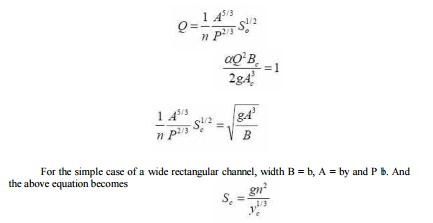Home | | Applied Hydraulic Engineering | Gradually varied flow

## Chapter: civil : Applied Hydraulic Engineering: Gradually Varied Flow

It is the inclusion of the channel slope and friction that allow us to decide which of the two depths is correct.

In the previous section of rapidly varied flow little mention was made of losses due to friction or the influence of the bed slope. It was assumed that frictional losses were insignificant - this is reasonable because rapidly varied flow occurs over a very short distance. However when it comes to long distances they become very important, and as gradually varied flow occurs over long distances we will consider friction losses here.

In the section on specific energy it was noted that there are two depth possible in steady flow for a given discharge at any point in the channel. (One is super-critical the other depth sub-critical.) The solution of the Manning equation results in only one depth - the normal depth.

It is the inclusion of the channel slope and friction that allow us to decide which of the two depths is correct. i.e. the channel slope and friction determine whether the uniform flow in the channel is sub or super-critical.

The procedure is

i.   Calculate the normal depth from Manning's equation

ii.   Calculate the critical depth from equation

The normal depth may be greater, less than or equal to the critical depth.

For a given channel and roughness there is only one slope that will give the normal depth equal to the critical depth. This slope is known as the critical slope ( Sc ).

If the slope is less than Sc the normal depth will be greater than critical depth and the flow will be sub-critical flow. The slope is termed mild .

If the slope is greater than Sc the normal depth will be less than critical depth and the flow will be super-critical flow. The slope is termed steep .

Problem of critical slope calculation

We have Equation that gives normal depth and equation that given critical depth Rearranging these in terms of Q and equating givesStudy Material, Lecturing Notes, Assignment, Reference, Wiki description explanation, brief detail
civil : Applied Hydraulic Engineering: Gradually Varied Flow : Gradually varied flow |

Related Topics